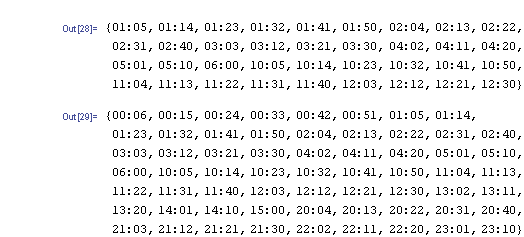# How Many Times In A 12 Hour Period Does The Sum Of The Digits On A Digital Clock Equal 6?

Oddman is correct as usual.  Contrary to my original answer, if it is a 12-hour digital clock, the answer is 36.  If it is a 24-hour clock, it will depend on the 12-hour period selected.
thanked the writer.
It depends on whether the clock displays seconds.
HH:MM
There are 36 instances on a 12-hour clock that displays hours 01-12.
There are 62 instances in 24 hours on a 24-hour clock that displays hours 00-23. 38 are before noon, 24 are after.
(see picture)

HH:MM:SS (same hour display as above)
There are 237 instances on a 12-hour clock.
There are 403 instances in 24 hours on a 24-hour clock.

Grellis is right: The answer for a 24-hour clock depends on the period selected.thanked the writer.